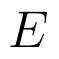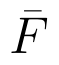Select Page

# 12 Science Maths CBSE Solutions for MCQ Differential Equations in English

12 Science Maths CBSE Solutions for MCQ Differential Equations in English to enable students to get Solutions in a narrative video format for the specific question.

Expert Teacher provides 12 Science Maths CBSE Solutions for MCQ Differential Equations through Video Solutions in English language. This video solution will be useful for students to understand how to write an answer in exam in order to score more marks. This teacher uses a narrative style for a question from Differential Equations not only to explain the proper method of answering question, but deriving right answer too.

Please find the question below and view the Solution in a narrative video format.

Question:

Solution Video in English:

You can select video Solutions from other languages also. Please check Solutions in ( Hindi )

## Similar Questions from CBSE, 12th Science, Maths, Differential Equations

Question 1 : Obtain the differential equation of the family of circles passing through the points (a, 0) and (-a, 0). (View Answer Video)

Question 3 : Write the degree of the differential equation(View Answer Video)

Question 4 :  Find the particular solution of the differential equationgiven thatWhen x =1.  (View Answer Video)

Question 5 : Find the general solution of the following differential equation :(View Answer Video)

### Application of Derivatives

Question 1 : The normal at the point (1, 1) on the curveis; (View Answer Video)

Question 2 : The normal to the curvepassing (1, 2) is : (View Answer Video)

Question 3 : A balloon, which always remains spherical on inflation, is being inflated by pumping in 900 cubic centimetres of gas per second. Find the rate at which the radius of the balloon increases when the radius is 15 cm. (View Answer Video)

Question 4 : The line y=mx+1 is a tangent to the curve ifthe value of m is ________. (View Answer Video)

Question 5 : The rate of change of the area of a circle with respect to its radius r at r = 6 cm is : (View Answer Video)

### Probability

Question 1 :  If E and F are independent events, then show thatandQuestion 4 : Ifandthen find(View Answer Video)